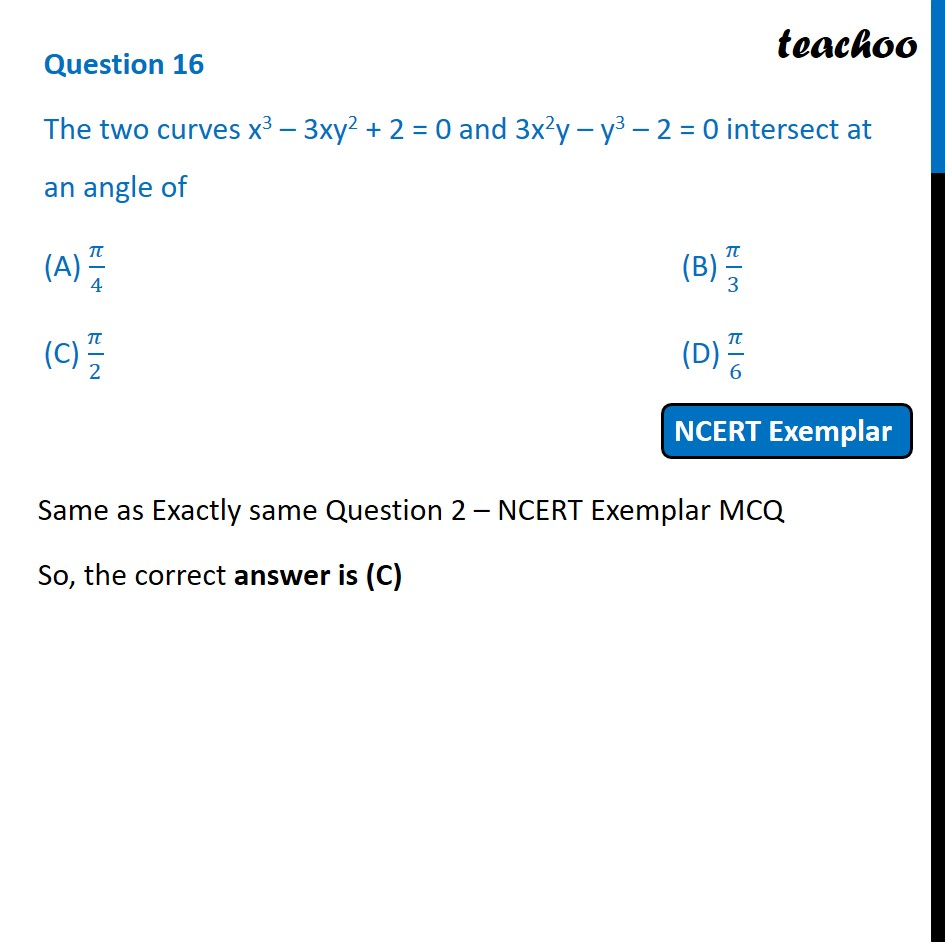NCERT Exemplar - MCQs

Chapter 6 Class 12 Application of Derivatives
Serial order wise

## (C) π/2             (D) π/6

This question is exactly same Question 2 NCERT Exemplar MCQ  - Chapter 6 Class 12 - Application of DerivativesLearn in your speed, with individual attention - Teachoo Maths 1-on-1 Class

### Transcript

Question 3 The two curves x3 – 3xy2 + 2 = 0 and 3x2y – y3 – 2 = 0 intersect at an angle of (A) 𝜋/4 (B) 𝜋/3 (C) 𝜋/2 (D) 𝜋/6 Same as Exactly same Question 2 – NCERT Exemplar MCQ So, the correct answer is (C)